# diffusebvarm

Bayesian vector autoregression (VAR) model with diffuse prior for data likelihood

## Description

The Bayesian VAR model object `diffusebvarm` specifies the joint prior distribution of the array of model coefficients Λ and the innovations covariance matrix Σ of an m-D VAR(p) model. The joint prior distribution (Λ,Σ) is the diffuse model.

A diffuse prior model does not enable you to specify hyperparameter values for coefficient sparsity; all AR lags in the model are weighted equally. To implement Minnesota regularization, create a conjugate, semiconjugate, or normal prior model by using `bayesvarm`.

In general, when you create a Bayesian VAR model object, it specifies the joint prior distribution and characteristics of the VARX model only. That is, the model object is a template intended for further use. Specifically, to incorporate data into the model for posterior distribution analysis, pass the model object and data to the appropriate object function.

## Creation

### Syntax

``PriorMdl = diffusebvarm(numseries,numlags)``
``PriorMdl = diffusebvarm(numseries,numlags,Name,Value)``

### Description

To create a `diffusebvarm` object, use either the `diffusebvarm` function (described here) or the `bayesvarm` function.

example

````PriorMdl = diffusebvarm(numseries,numlags)` creates a `numseries`-D Bayesian VAR(`numlags`) model object `PriorMdl`, which specifies dimensionalities and prior assumptions for all model coefficients $\lambda =\text{vec}\left(\Lambda \right)=\text{vec}\left({\left[\begin{array}{ccccccc}{\Phi }_{1}& {\Phi }_{2}& \cdots & {\Phi }_{p}& c& \delta & Β\end{array}\right]}^{\prime }\right)$ and the innovations covariance Σ, where: `numseries` = m, the number of response time series variables.`numlags` = p, the AR polynomial order.The joint prior distribution of (λ,Σ) is the diffuse model. ```

example

``PriorMdl = diffusebvarm(numseries,numlags,Name,Value)` sets writable properties (except `NumSeries` and `P`) using name-value pair arguments. Enclose each property name in quotes. For example, `diffusebvarm(3,2,'SeriesNames',["UnemploymentRate" "CPI" "FEDFUNDS"])` specifies the names of the three response variables in the Bayesian VAR(2) model.`

### Input Arguments

expand all

Number of time series m, specified as a positive integer. `numseries` specifies the dimensionality of the multivariate response variable yt and innovation εt.

`numseries` sets the `NumSeries` property.

Data Types: `double`

Number of lagged responses in each equation of yt, specified as a nonnegative integer. The resulting model is a VAR(`numlags`) model; each lag has a `numseries`-by-`numseries` coefficient matrix.

`numlags` sets the `P` property.

Data Types: `double`

## Properties

expand all

You can set writable property values when you create the model object by using name-value pair argument syntax, or after you create the model object by using dot notation. For example, to create a 3-D Bayesian VAR(1) model and label the first through third response variables, and then include a linear time trend term, enter:

```PriorMdl = diffusebvarm(3,1,'SeriesNames',["UnemploymentRate" "CPI" "FEDFUNDS"]); PriorMdl.IncludeTrend = true;```

## Model Characteristics and Dimensionality

Model description, specified as a string scalar or character vector. The default value describes the model dimensionality, for example `'2-Dimensional VAR(3) Model'`.

Example: `"Model 1"`

Data Types: `string` | `char`

This property is read-only.

Number of time series m, specified as a positive integer. `NumSeries` specifies the dimensionality of the multivariate response variable yt and innovation εt.

Data Types: `double`

This property is read-only.

Multivariate autoregressive polynomial order, specified as a nonnegative integer. `P` is the maximum lag that has a nonzero coefficient matrix.

`P` specifies the number of presample observations required to initialize the model.

Data Types: `double`

Response series names, specified as a `NumSeries` length string vector. The default is `['Y1' 'Y2' ... 'YNumSeries']`. `diffusebvarm` stores `SeriesNames` as a string vector.

Example: `["UnemploymentRate" "CPI" "FEDFUNDS"]`

Data Types: `string`

Flag for including a model constant c, specified as a value in this table.

ValueDescription
`false`Response equations do not include a model constant.
`true`All response equations contain a model constant.

Data Types: `logical`

Flag for including a linear time trend term δt, specified as a value in this table.

ValueDescription
`false`Response equations do not include a linear time trend term.
`true`All response equations contain a linear time trend term.

Data Types: `logical`

Number of exogenous predictor variables in the model regression component, specified as a nonnegative integer. `diffusebvarm` includes all predictor variables symmetrically in each response equation.

## VAR Model Parameters Derived from Distribution Hyperparameters

This property is read-only.

Distribution mean of the autoregressive coefficient matrices Φ1,…,Φp associated with the lagged responses, specified as a `P`-D cell vector of `NumSeries`-by-`NumSeries` numeric matrices.

`AR{j}` is Φ`j`, the coefficient matrix of lag `j` . Rows correspond to equations and columns correspond to lagged response variables; `SeriesNames` determines the order of response variables and equations. Coefficient signs are those of the VAR model expressed in difference-equation notation.

If `P` = 0, `AR` is an empty cell. Otherwise, `AR` is the collection of AR coefficient means extracted from `Mu`.

Data Types: `cell`

This property is read-only.

Distribution mean of the model constant c (or intercept), specified as a `NumSeries`-by-1 numeric vector. `Constant(j)` is the constant in equation `j`; `SeriesNames` determines the order of equations.

If `IncludeConstant` = `false`, `Constant` is an empty array. Otherwise, `Constant` is the model constant vector mean extracted from `Mu`.

Data Types: `double`

This property is read-only.

Distribution mean of the linear time trend δ, specified as a `NumSeries`-by-1 numeric vector. `Trend(j)` is the linear time trend in equation `j`; `SeriesNames` determines the order of equations.

If `IncludeTrend` = `false` (the default), `Trend` is an empty array. Otherwise, `Trend` is the linear time trend coefficient mean extracted from `Mu`.

Data Types: `double`

This property is read-only.

Distribution mean of the regression coefficient matrix B associated with the exogenous predictor variables, specified as a `NumSeries`-by-`NumPredictors` numeric matrix.

`Beta(j,:)` contains the regression coefficients of each predictor in the equation of response variable j y`j`,t. `Beta(:,k)` contains the regression coefficient in each equation of predictor xk. By default, all predictor variables are in the regression component of all response equations. You can down-weight a predictor from an equation by specifying, for the corresponding coefficient, a prior mean of 0 in `Mu` and a small variance in `V`.

When you create a model, the predictor variables are hypothetical. You specify predictor data when you operate on the model (for example, when you estimate the posterior by using `estimate`). Columns of the predictor data determine the order of the columns of `Beta`.

Data Types: `double`

Distribution mean of the innovations covariance matrix Σ of the `NumSeries` innovations at each time t = 1,...,T, specified as a `NumSeries`-by-`NumSeries` matrix of `NaN`. Because the prior model is diffuse, the mean of Σ is unknown, a priori.

## Object Functions

 `estimate` Estimate posterior distribution of Bayesian vector autoregression (VAR) model parameters `forecast` Forecast responses from Bayesian vector autoregression (VAR) model `simsmooth` Simulation smoother of Bayesian vector autoregression (VAR) model `simulate` Simulate coefficients and innovations covariance matrix of Bayesian vector autoregression (VAR) model `summarize` Distribution summary statistics of Bayesian vector autoregression (VAR) model

## Examples

collapse all

Consider the 3-D VAR(4) model for the US inflation (`INFL`), unemployment (`UNRATE`), and federal funds (`FEDFUNDS`) rates.

`$\left[\begin{array}{l}{\text{INFL}}_{t}\\ {\text{UNRATE}}_{t}\\ {\text{FEDFUNDS}}_{t}\end{array}\right]=c+\sum _{j=1}^{4}{\Phi }_{j}\left[\begin{array}{l}{\text{INFL}}_{t-j}\\ {\text{UNRATE}}_{t-j}\\ {\text{FEDFUNDS}}_{t-j}\end{array}\right]+\left[\begin{array}{c}{\epsilon }_{1,t}\\ {\epsilon }_{2,t}\\ {\epsilon }_{3,t}\end{array}\right].$`

For all $t$, ${\epsilon }_{t}$ is a series of independent 3-D normal innovations with a mean of 0 and covariance $\Sigma$. Assume that the joint prior distribution of the VAR model parameters $\left({\left[{\Phi }_{1},...,{\Phi }_{4},\mathit{c}\right]}^{\prime },\Sigma \right)$ is diffuse.

Create a diffuse prior model for the 3-D VAR(4) model parameters.

```numseries = 3; numlags = 4; PriorMdl = diffusebvarm(numseries,numlags)```
```PriorMdl = diffusebvarm with properties: Description: "3-Dimensional VAR(4) Model" NumSeries: 3 P: 4 SeriesNames: ["Y1" "Y2" "Y3"] IncludeConstant: 1 IncludeTrend: 0 NumPredictors: 0 AR: {[3x3 double] [3x3 double] [3x3 double] [3x3 double]} Constant: [3x1 double] Trend: [3x0 double] Beta: [3x0 double] Covariance: [3x3 double] ```

`PriorMdl` is a `diffusebvarm` Bayesian VAR model object representing the prior distribution of the coefficients and innovations covariance of the 3-D VAR(4) model. The command line display shows properties of the model. You can display properties by using dot notation.

Display the prior covariance mean matrices of the four AR coefficients by setting each matrix in the cell to a variable.

`AR1 = PriorMdl.AR{1}`
```AR1 = 3×3 0 0 0 0 0 0 0 0 0 ```
`AR2 = PriorMdl.AR{2}`
```AR2 = 3×3 0 0 0 0 0 0 0 0 0 ```
`AR3 = PriorMdl.AR{3}`
```AR3 = 3×3 0 0 0 0 0 0 0 0 0 ```
`AR4 = PriorMdl.AR{4}`
```AR4 = 3×3 0 0 0 0 0 0 0 0 0 ```

`diffusebvarm` centers all AR coefficients at `0` by default. Because the model is diffuse, the data informs the posterior distribution.

Consider a 1-D Bayesian AR(2) model for the daily NASDAQ returns from January 2, 1990 through December 31, 2001.

`${y}_{t}=c+{\varphi }_{1}{y}_{t-1}+{\varphi }_{2}{y}_{t-1}+{\epsilon }_{t}.$`

The joint prior is diffuse.

Create a diffuse prior model for the AR(2) model parameters.

```numseries = 1; numlags = 2; PriorMdl = diffusebvarm(numseries,numlags)```
```PriorMdl = diffusebvarm with properties: Description: "1-Dimensional VAR(2) Model" NumSeries: 1 P: 2 SeriesNames: "Y1" IncludeConstant: 1 IncludeTrend: 0 NumPredictors: 0 AR: { } Constant: 0 Trend: [1x0 double] Beta: [1x0 double] Covariance: NaN ```

Consider adding a linear time trend term to the 3-D VAR(4) model of Create Diffuse Prior Model:

`$\left[\begin{array}{l}{\text{INFL}}_{t}\\ {\text{UNRATE}}_{t}\\ {\text{FEDFUNDS}}_{t}\end{array}\right]=c+\delta t+\sum _{j=1}^{4}{\Phi }_{j}\left[\begin{array}{l}{\text{INFL}}_{t-j}\\ {\text{UNRATE}}_{t-j}\\ {\text{FEDFUNDS}}_{t-j}\end{array}\right]+\left[\begin{array}{c}{\epsilon }_{1,t}\\ {\epsilon }_{2,t}\\ {\epsilon }_{3,t}\end{array}\right].$`

Create a diffuse prior model for the 3-D VAR(4) model parameters. Specify response variable names.

```numseries = 3; numlags = 4; seriesnames = ["INFL"; "UNRATE"; "FEDFUNDS"]; PriorMdl = diffusebvarm(numseries,numlags,'SeriesNames',seriesnames,... 'IncludeTrend',true)```
```PriorMdl = diffusebvarm with properties: Description: "3-Dimensional VAR(4) Model" NumSeries: 3 P: 4 SeriesNames: ["INFL" "UNRATE" "FEDFUNDS"] IncludeConstant: 1 IncludeTrend: 1 NumPredictors: 0 AR: {[3x3 double] [3x3 double] [3x3 double] [3x3 double]} Constant: [3x1 double] Trend: [3x1 double] Beta: [3x0 double] Covariance: [3x3 double] ```

Consider the 2-D VARX(1) model for the US real GDP (`RGDP`) and investment (`GCE`) rates that treats the personal consumption (`PCEC`) rate as exogenous:

`$\left[\begin{array}{l}{\text{RGDP}}_{t}\\ {\text{GCE}}_{t}\end{array}\right]=c+\Phi \left[\begin{array}{l}{\text{RGDP}}_{t-1}\\ {\text{GCE}}_{t-1}\end{array}\right]+{\text{PCEC}}_{t}\beta +{\epsilon }_{t}.$`

For all $t$, ${\epsilon }_{t}$ is a series of independent 2-D normal innovations with a mean of 0 and covariance $\Sigma$. Assume that the joint prior distribution is diffuse.

Create a diffuse prior model for the 2-D VARX(1) model parameters.

```numseries = 2; numlags = 1; numpredictors = 1; PriorMdl = diffusebvarm(numseries,numlags,'NumPredictors',numpredictors)```
```PriorMdl = diffusebvarm with properties: Description: "2-Dimensional VAR(1) Model" NumSeries: 2 P: 1 SeriesNames: ["Y1" "Y2"] IncludeConstant: 1 IncludeTrend: 0 NumPredictors: 1 AR: {[2x2 double]} Constant: [2x1 double] Trend: [2x0 double] Beta: [2x1 double] Covariance: [2x2 double] ```

Consider the 3-D VAR(4) model of Create Diffuse Prior Model. Estimate the posterior distribution, and generate forecasts from the corresponding posterior predictive distribution.

Load and Preprocess Data

Load the US macroeconomic data set. Compute the inflation rate. Plot all response series.

```load Data_USEconModel seriesnames = ["INFL" "UNRATE" "FEDFUNDS"]; DataTimeTable.INFL = 100*[NaN; price2ret(DataTimeTable.CPIAUCSL)]; figure plot(DataTimeTable.Time,DataTimeTable{:,seriesnames}) legend(seriesnames)```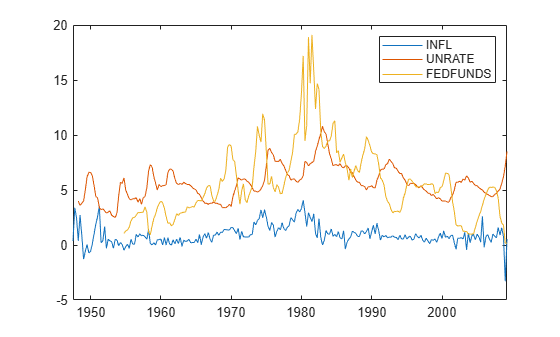Stabilize the unemployment and federal funds rates by applying the first difference to each series.

```DataTimeTable.DUNRATE = [NaN; diff(DataTimeTable.UNRATE)]; DataTimeTable.DFEDFUNDS = [NaN; diff(DataTimeTable.FEDFUNDS)]; seriesnames(2:3) = "D" + seriesnames(2:3);```

Remove all missing values from the data.

`rmDataTimeTable = rmmissing(DataTimeTable);`

Create Prior Model

Create a diffuse Bayesian VAR(4) prior model for the three response series. Specify the response variable names.

```numseries = numel(seriesnames); numlags = 4; PriorMdl = diffusebvarm(numseries,numlags,'SeriesNames',seriesnames);```

Estimate Posterior Distribution

Estimate the posterior distribution by passing the prior model and entire data series to `estimate`.

```rng(1); % For reproducibility PosteriorMdl = estimate(PriorMdl,rmDataTimeTable{:,seriesnames},'Display','equation');```
```Bayesian VAR under diffuse priors Effective Sample Size: 197 Number of equations: 3 Number of estimated Parameters: 39 VAR Equations | INFL(-1) DUNRATE(-1) DFEDFUNDS(-1) INFL(-2) DUNRATE(-2) DFEDFUNDS(-2) INFL(-3) DUNRATE(-3) DFEDFUNDS(-3) INFL(-4) DUNRATE(-4) DFEDFUNDS(-4) Constant ------------------------------------------------------------------------------------------------------------------------------------------------------------------------------ INFL | 0.1241 -0.4809 0.1005 0.3236 -0.0503 0.0450 0.4272 0.2738 0.0523 0.0167 -0.1830 0.0067 0.1007 | (0.0762) (0.1536) (0.0390) (0.0868) (0.1647) (0.0413) (0.0860) (0.1620) (0.0428) (0.0901) (0.1520) (0.0395) (0.0832) DUNRATE | -0.0219 0.4716 0.0391 0.0913 0.2414 0.0536 -0.0389 0.0552 0.0008 0.0285 -0.1795 0.0088 -0.0499 | (0.0413) (0.0831) (0.0211) (0.0469) (0.0891) (0.0223) (0.0465) (0.0876) (0.0232) (0.0488) (0.0822) (0.0214) (0.0450) DFEDFUNDS | -0.1586 -1.4368 -0.2905 0.3403 -0.2968 -0.3117 0.2848 -0.7401 0.0028 -0.0690 0.1494 -0.1372 -0.4221 | (0.1632) (0.3287) (0.0835) (0.1857) (0.3526) (0.0883) (0.1841) (0.3466) (0.0917) (0.1928) (0.3253) (0.0845) (0.1781) Innovations Covariance Matrix | INFL DUNRATE DFEDFUNDS ------------------------------------------- INFL | 0.3028 -0.0217 0.1579 | (0.0321) (0.0124) (0.0499) DUNRATE | -0.0217 0.0887 -0.1435 | (0.0124) (0.0094) (0.0283) DFEDFUNDS | 0.1579 -0.1435 1.3872 | (0.0499) (0.0283) (0.1470) ```

`PosteriorMdl` is a `conjugatebvarm` model object; the posterior is analytically tractable. By default, `estimate` uses the first four observations as a presample to initialize the model.

Generate Forecasts from Posterior Predictive Distribution

From the posterior predictive distribution, generate forecasts over a two-year horizon. Because sampling from the posterior predictive distribution requires the entire data set, specify the prior model in `forecast` instead of the posterior.

```fh = 8; FY = forecast(PriorMdl,fh,rmDataTimeTable{:,seriesnames});```

`FY` is an 8-by-3 matrix of forecasts.

Plot the end of the data set and the forecasts.

```fp = rmDataTimeTable.Time(end) + calquarters(1:fh); figure plotdata = [rmDataTimeTable{end - 10:end,seriesnames}; FY]; plot([rmDataTimeTable.Time(end - 10:end); fp'],plotdata) hold on plot([fp(1) fp(1)],ylim,'k-.') legend(seriesnames) title('Data and Forecasts') hold off```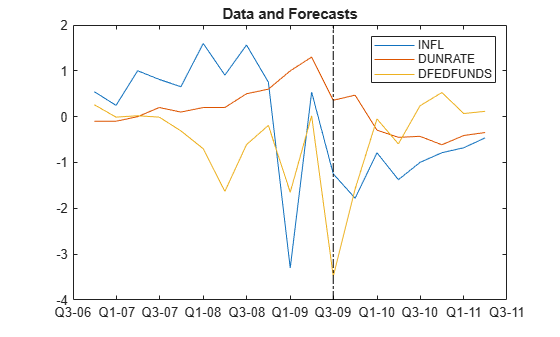Compute Impulse Responses

Plot impulse response functions by passing posterior estimations to `armairf`.

`armairf(PosteriorMdl.AR,[],'InnovCov',PosteriorMdl.Covariance)`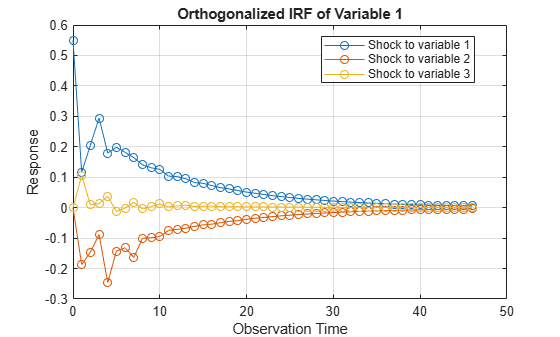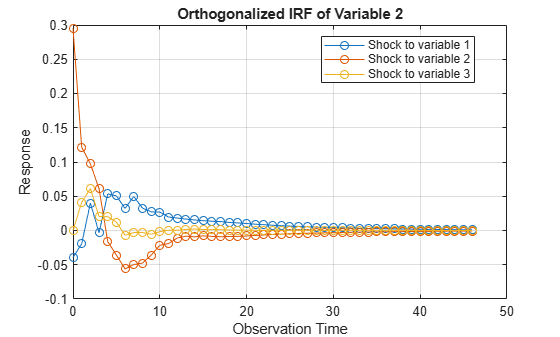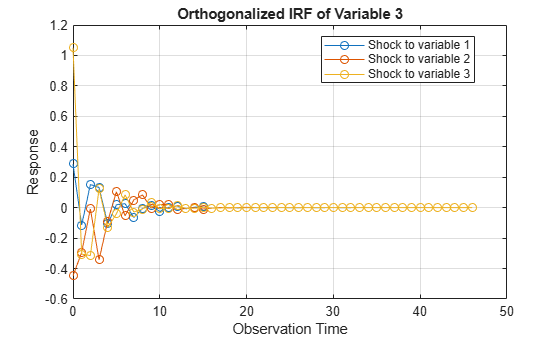expand all

## Version History

Introduced in R2020a